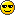# Met Exam - the Fohn Wind Effect##Met Exam - the Fohn Wind Effect

Hi,

I'm getting stuck with the following question on airquiz:

At sea level on the windward side of the mountain, the air has a temperature of +19 and dew point +5 degrees Celsius. Approximately what temperature would you expect the air to be on the lee side at 2000 ft? (Mountain is 4000ft high)

Answer: 13 degrees Celsius

I can work out the cloud base on the windward side: (19-5)/3 *1000 =~4600 ft.

How is this converted to a temperature on the lee side?

Any help appreciated!

Cheers,
Peter

Peter

Posts : 4
Join date : 2010-03-19

##Re: Met Exam - the Fohn Wind Effect

Hi,

For information the answer (from AirQuiz) is as follows.

1) Sea level = 19 deg C.
2) At 1000ft temp = 19 – 3 = 16 (using the DALR)
3) At 2000ft temp = 16 -3 = 13
4) At 3000ft temp = 13-3 = 10
5) At 4000ft (summit of mountain) temp = 10-3 = 7
6) Note we have not hit the dewpoint of 5 deg C in this question so use of DALR continues.
7) Descending the other side we drop to 3000ftAt 3000ft temp = 7 + 3 = 10
9) At 2000ft temp = 10 + 3 = 13 deg C.

If the dewpoint was reached on the windward side, SALR would be required. An estimate of the dewpoint would be needed on the other side. However for this question to work, the dewpoint temp will not be reached.

Cheers,
Peter

Peter

Posts : 4
Join date : 2010-03-19Permissions in this forum:
You cannot reply to topics in this forum